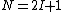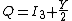# i-spin

## I-spin

A quantum-mechanical variable or quantum number applied to quarks and their compounds, the strongly interacting fundamental hadrons, and the compounds of those hadrons (such as nuclear states) to facilitate consideration of the consequences of the charge independence of the strong (nuclear) forces. This variable is also known as isotopic spin, isobaric spin, and isospin.

The many strongly interacting particles (hadrons) and the compounds of these particles, such as nuclei, are observed to form sets or multiplets such that the members of the multiplet differ in their electric charge and magnetic moments, and other electromagnetic properties but are otherwise almost identical. For example, the neutron and proton, with electric charges that are zero and plus one (in units of the magnitude of the electron charge), form a set of two such states. The pions, one with a unit of positive charge, one with zero charge, and one with a unit of negative charge, form a set of three. It appears that if the effects of electromagnetic forces and the closely related weak nuclear forces (responsible for beta decay) are neglected, leaving only the strong forces effective, the different members of such a multiplet are equivalent and cannot be distinguished in their strong interactions. The strong interactions are thus independent of the different electric charges held by different members of the set; they are charge-independent. See Elementary particle, Fundamental interactions, Hadron, Strong nuclear interactions

The I-spin (I) of such a set or multiplet of equivalent states is defined such that Eq. (1)

(1)is satisfied, where N is the number of states in the set. Another quantum number I3, called the third component of I-spin, is used to differentiate the numbers of a multiplet where the values of I3 vary from +I to -I in units of one. The charge Q of a state and the value of I3 for
(2)this state are connected by the Gell-Mann–Okubo relation (2), where Y, the charge offset, is called hypercharge. For nuclear states, Y is simply the number of nucleons. Electric charge is conserved in all interactions; Y is observed to be conserved by the strong forces so that I3 is conserved in the course of interactions mediated by the strong forces. See Hypercharge

This description of a multiplet of states with I-spin is similar to the quantum-mechanical description of a particle with a total angular momentum or spin of j (in units of &planck;, Planck's constant divided by 2&pgr;). Such a particle can be considered as a set of states which differ in their orientation or component of spin jz in a z direction of quantization. There are 2j + 1 such states, where jz varies from -j to +j in steps of one unit. To the extent that the local universe is isotropic (or there are no external forces on the states that depend upon direction), the components of angular momentum in any direction are conserved, and states with different values of jz are dynamically equivalent.

There is then a logical or mathematical equivalence between the descriptions of (1) a multiplet of states of definite I and different values of I3 with respect to charge-independent forces and (2) a multiplet of states of a particle with a definite spin j and different values of jz with respect to direction-independent forces. In each case, the members of the multiplet with different values of the conserved quantity I3 on the one hand and jz on the other are dynamically equivalent; that is, they are indistinguishable by any application of the forces in question. See Angular momentum, Spin (quantum mechanics)

The charge independence of the strong interactions has important consequences, defining the intensity ratios of different charge states produced in those particle reactions and decays which are mediated by the strong interactions. I-spin considerations also provide insight into the total energies or masses of nuclear and particle states. The basis for the symmetry described by I-spin is to be found in the quark structure of the strongly interacting particles and the character of the interactions between quarks. See Quarks, Selection rules (physics), Symmetry laws (physics)

McGraw-Hill Concise Encyclopedia of Physics. © 2002 by The McGraw-Hill Companies, Inc.

## i-spin

[′ī ‚spin]
(nuclear physics)
McGraw-Hill Dictionary of Scientific & Technical Terms, 6E, Copyright © 2003 by The McGraw-Hill Companies, Inc.
References in periodicals archive ?
'So political convenience 'yan na gagamitin nila, i-spin nila na parang may anti-corruption drive na nangyayari,' he said of Sueno's dismissal.

Site: Follow: Share:
Open / Close